• **对于变量有二个以上的情况，贝式定理亦成立。...首先大家都知道一个耳熟能详的条件概率公式P(A|B)=P(A,B)/P(B),那么我们可以将B,C同时发生记为事件T,所以P(A|T)=P(A,T)/P(T)。则有： P(A|B,C)=P(A,B,C)/P(B...
对于变量有二个以上的情况，贝式定理亦成立。P(A|B,C)=P(B|A)*P(A)*M / ( P(B)*P(C|B) ).则M=()。 我将通过求出M的方式来告诉大家多变量条件概率公式如何推导。 首先大家都知道一个耳熟能详的条件概率公式P(A|B)=P(A,B)/P(B),那么我们可以将B,C同时发生记为事件T,所以P(A|T)=P(A,T)/P(T)。则有：P(A|B,C)=P(A,B,C)/P(B,C) 1、 P(A,B,C)=P(C,A,B)=P(C|A,B)*P(A,B)=P(C|A,B)*P(B,A)=P(C|A,B)*P(B|A)*P(A) 2、 P(B,C)=P(C|B)*P(B) 综上所述：M=P(C|A,B) 由上述求解M的过程可知，M的结果跟其分子分母的形式有关，所以多变量情况下贝叶斯定理依然成立但是形式不唯一。
展开全文• 原文来自师兄的博客：...多元正态分布的条件密度 多元正态分布多元正态分布的密度函数如下 ： fx(x1,...xn)=1(2π)k√|Σ|1/2exp(−12(x−μ)TΣ−1(x−μ))f_{x}(x_{1},...x_{n})=\frac{1}{\sqrt{(2\pi)^{
原文来师兄的博客：http://blog.csdn.net/wjj5881005/article/details/53320403

多元正态分布多元正态分布的条件密度

多元正态分布
多元正态分布的密度函数如下 ：

fx(x1,...xn)=1(2π)k√|Σ|1/2exp(−12(x−μ)TΣ−1(x−μ))
$f_{x}(x_{1},...x_{n})=\frac{1}{\sqrt{(2\pi)^{k}}|\Sigma|^{1/2}}exp(-\frac{1}{2}(x-\mu)^{T}\Sigma^{-1}(x-\mu))$ (1)

其对应的矩母函数（也有称动差函数）为

exp(μTt+12tTΣt)
$exp(\mu^{T}t+\frac{1}{2}t^{T}\Sigma t)$。事实上，如果随机向量

[X1,...Xn]
$[X_{1},...X_{n}]$满足上面的动差函数，那么我们就称随机向量

[X1,...Xn]
$[X_{1},...X_{n}]$服从多元高斯分布。具体地证明可以看
这里。

多元正态分布的条件密度
令随机向量

[X1,...Xn]
$[X_{1},...X_{n}]$服从多元高斯分布。我们可以推导

Xn
$X_{n}$在给定

X1,...Xn−1
$X_{1},...X_{n-1}$的情况下的条件密度分布：

f(xn|x1,...,xn−1)=f(x1,...,xn−1,xn)f(x1,...,xn−1)
$f(x_{n}|x_{1},...,x_{n-1})=\frac{f(x_{1},...,x_{n-1},x_{n})}{f(x_{1},...,x_{n-1})}$ (2)，

其中

f(x1,...,xn)=(2π)−n/2(|Σ|−1/2)exp[−12∑ni,j=1yiqijyj]
$f(x_{1},...,x_{n})=(2\pi)^{-n/2}(|\Sigma|^{-1/2})exp[-\frac{1}{2}\sum_{i,j=1}^{n}y_{i}q_{ij}y_{j}]$ (3)

其中

Q=Σ−1=[qij],yi=xi−μi
$Q=\Sigma^{-1}=[q_{ij}],y_{i}=x_{i}-\mu_{i}$。同样地，

f(x1,...,xn−1)=∫∞∞f(x1,...,xn−1,xn)dxn=B(y1,...,yn−1)
$f(x_{1},...,x_{n-1})=\int_\infty^\infty {f(x_{1},...,x_{n-1},x_{n})}\,dx_{n}=B(y_{1},...,y_{n-1})$ (4).

现在我们将公式(3)中的求和项进行分解，有：

∑ni,j=1yiqijyj=∑n−1i,j=1yiqijyj+yn∑n−1j=1qnjyj+yn∑n−1i=1qinyj+qnny2n
$\sum_{i,j=1}^{n}y_{i}q_{ij}y_{j}=\sum_{i,j=1}^{n-1}y_{i}q_{ij}y_{j}+y_{n}\sum_{j=1}^{n-1}q_{nj}y_{j}+y_{n}\sum_{i=1}^{n-1}q_{in}y_{j}+q_{nn}y_{n}^{2}$(5)

因此，最终地条件分布具有如下的形式：

A(y1,...,yn−1)B(y1,...,yn−1)exp[−(Cy2n+D(y1,...,yn−1)yn)]
$\frac{A(y_{1},...,y_{n-1})}{B(y_{1},...,y_{n-1})}exp[-(Cy_{n}^{2}+D(y_{1},...,y_{n-1})y_{n})]$ (6)

其中

C=(1/2)qnn
$C=(1/2)q_{nn}$，因为

Q=Σ−1
$Q=\Sigma^{-1}$是对称矩阵，所以

D=∑n−1j=1qnjyj=∑n−1i=1qinyi
$D=\sum_{j=1}^{n-1}q_{nj}y_{j}=\sum_{i=1}^{n-1}q_{in}y_{i}$.(6)式又可以进一步表示称如下的式子：

[ABexp(DD24C)]exp[−(yn+D2C)2]1C
$[\frac{A}{B}exp(\frac{DD^{2}}{4C})]exp[-\frac{(y_{n}+\frac{D}{2C})^{2}]}{\frac{1}{C}}$ (7)

从公式(7)很容看出

xn
$x_{n}$的条件密度函数是服从正态分布的。
所以条件分布的方差为：

2Var(Xn|X1,...,Xn−1)=1/C
$2Var(X_{n}|X_{1},...,X_{n-1})=1/C$，进一步有：

Var(Xn|X1,...,Xn−1)=12C=1qnn
$Var(X_{n}|X_{1},...,X_{n-1})=\frac{1}{2C}=\frac{1}{q_{nn}}$
均值为：

E(Xn|X1,...,Xn−1)=μn−D2C=μn−1qnn∑n−1j=1qnj(Xj−μj)
$E(X_{n}|X_{1},...,X_{n-1})=\mu_{n}-\frac{D}{2C}=\mu_{n}-\frac{1}{q_{nn}}\sum_{j=1}^{n-1}q_{nj}(X_{j}-\mu_{j})$

这就说明了再抽样多元正态分布时，如果已知了其它维度的随机变量值，
剩下的那个维度的随机变量也是服从正态分布。

展开全文• 相信你对变量这个概念并不陌生，数学方程式和编程代码里经常会用到变量。那什么是变量呢？我们在概率中常说的随机变量（ random variable）和普通的变量（variable）又有什么...

相信你对变量这个概念并不陌生，数学方程式和编程代码里经常会用到变量。那什么是变量呢？我们在概率中常说的随机变量（ random variable）和普通的变量（variable）又有什么不同呢？
这些问题其实很简单，我一说你就明白了。
在没有发生运算之前，普通变量的值并不会发生变化，也就是说，它可以取不同的值，但是一旦取值确定之后，它总会是一个固定的值，除非有新的运算操作。而随机变量的值并不固定，比如说，某个随机变量可能有 10% 的概率等于 10，有 20% 的概率等于 5，有 30% 的概率等于 28 等等。
我们上节说了，随机变量根据其取值是否连续，可分为离散型随机变量和连续型随机变量。举几个例子，抛硬币出现正反面的次数以及每周下雨的天数，都是离散的值，所以对应的随机变量为离散型。而汽车每小时行驶的速度和银行排队的时间，都是连续的值，对应的随机变量为连续型。换句话，从计算的角度来说，我们可以直接求和得出的，就是“离散的”，需要用积分计算的，就是“连续的”。
而随机变量的取值对应了随机现象的一种结果。正是结果的不确定性，才导致了随机变量取值的不确定性，于是我们就引入了概率。我们可以说，每种值是以一定的概率出现的。
概率分布
随机变量的每种取值的出现都遵从一定的可能性，把这个可能性用具体的数值表示出来就是概率。如果将随机变量所有可能出现的值，及其对应的概率都罗列出来，我们就能获得这个变量的概率分布。
我们拿最简单的抛硬币事件来看。从理论上说来，出现正面和反面的概率都是 50%（我们假设不存在硬币站立的情况）。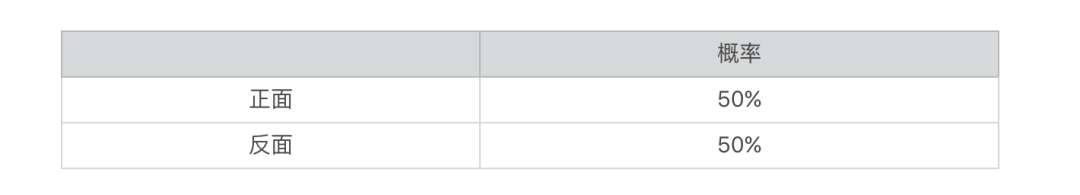我们可以通过一小段 Python 代码，做个模拟实验，验证一下这个分布。
import random
import pandas as pd
import numpy as np
import matplotlib.pyplot as plt

def flip_coin(times):
data_array = np.empty(times)
weights_array = np.empty(times)
weights_array.fill(1 / times)

for i in range(0, times): #抛times次的硬币
data_array[i] = random.randint(0, 1) #假设0表示正面，1表示反面

data_frame = pd.DataFrame(data_array)
data_frame.plot(kind = 'hist', legend = False) #获取正反面统计次数的直方图
data_frame.plot(kind = 'hist', legend = False, weights = weights_array).set_ylabel("Probability") #获取正反面统计概率的直方图
plt.show()

flip_coin(10)
该代码随机生成若干次的 0 或 1（0 表示硬币正面朝上，1 表示硬币反面朝上）。下表是我生成 10 次的结果，其中正面 4 次，反面 6 次。对应的正反面次数和概率直方图如下，而概率的直方图就表示了其概率分布。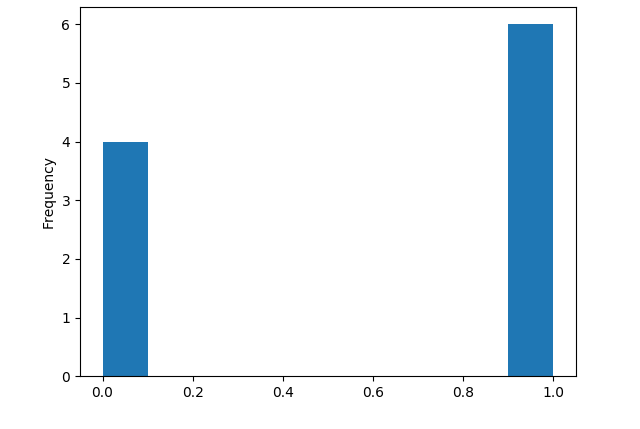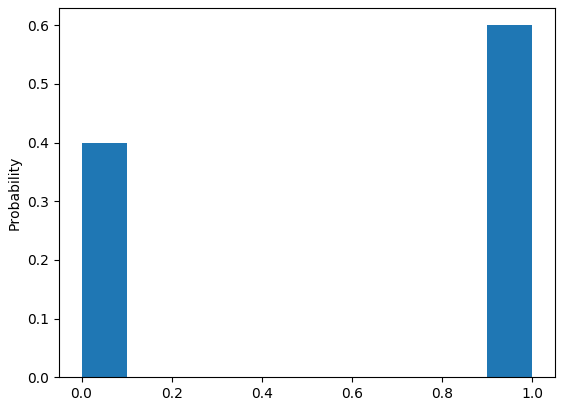通过修改 flip_coin 函数中的数字，我们可以修改抛硬币的次数。我又尝试“抛了”100 次，得到的结果是正面 47 次，反面 53 次。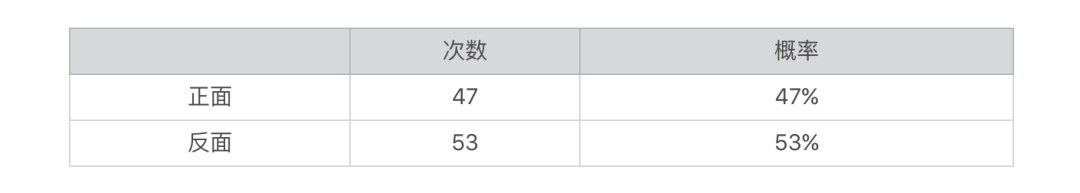接下来是抛 10000 次的结果，正面是 4982 次，反面是 5018 次。你可能已经发现了，根据计算机模拟的结果所统计的概率，并不是精确的正反面各 50%。如果你运行同样的代码，也会发现类似的情况。这是因为理论上的概率，是基于无限次的实验。而我们这里实验的次数是有限的，是一种统计采样。
从 10 次、100 次到 10000 次，我们能看到，概率会变得越来越稳定，越来越趋近于正反各 50% 的分布。也就是说，统计的采样次数越多，越趋近于我们理论上的情况。因此，从这个统计实验我们可以看出，概率分布描述的其实就是随机变量的概率规律。
抛硬币正面次数、每周下雨天数这种离散型随机变量，对应的概率分布是很好理解的，但是对于连续型的随机变量，如何理解它们的概率分布呢？
如果我们把连续的值离散化，你会发现这个问题其实不难理解。就拿汽车每小时行驶的公里数来说吧。现实生活中我们通过汽车的仪表盘所读取速度，都是整数值，例如每小时 60 公里。也许比较高档的车会显示数字化的速度，带有小数位，但实际上汽车最精确的速度是一个无限位数的小数，是从 0 到最高公里数的一个任意数值。所以仪表盘所显示的数字，是将实际速度离散化处理之后的数字。除了仪表盘上的速度，汽车行驶在时间维度上也是连续的。类似地，我们还需要对时间进行离散化，比如每分钟查看仪表盘一次并读取速度值。
好的，理解了这些之后，我同样使用代码来随机一些行驶速度的数据。第一次模拟，假设我们手头上有一辆老爷车，它的仪表盘最小刻度是 5，也就是说，它只能显示 55、60、65 这种公里数。然后我们每 1 分钟采样一次（读一次仪表盘），那么 1 小时内我们将生成 60 个数据。示例代码如下：
def check_speed(time_gap, speed_gap, total_time, min_speed, max_speed):

times = (int)(total_time / time_gap) #获取读取仪表盘的次数

data_array = np.empty(times)
weights_array = np.empty(times)
weights_array.fill(1 / times)

for i in range(0, times):
if (speed_gap < 1):
data_array[i] = random.random() * max_speed #随机生成一个最高速和最低速之间的速度
else:
data_array[i] = random.randint(0, max_speed / speed_gap) * speed_gap #随机生成一个最高速和最低速之间的速度，先除以speed_gap然后乘以speed_gap进行离散化

data_frame = pd.DataFrame(data_array)
bin_range = np.arange(0, 200, speed_gap)
data_frame.plot(kind = 'hist', bins = bin_range, legend = False) #获取时速统计次数的直方图
data_frame.plot(kind = 'hist', bins = bin_range, legend = False, weights = weights_array, ).set_ylabel("Probability") #获取时速统计概率的直方图
plt.show()

check_speed(1, 5, 60, 0, 200)
对生成的 60 个数据，我们统计其出现在每个速度区间的频次以及相应的概率。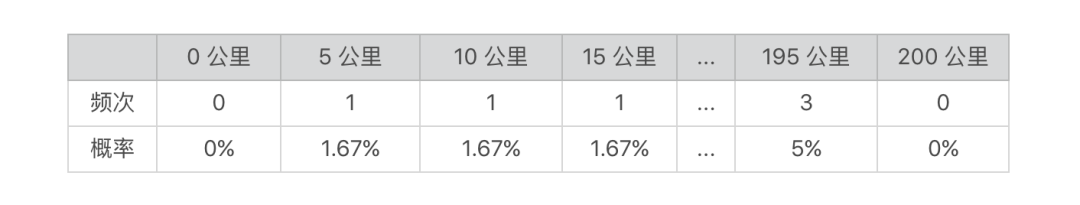我们将频次和概率以直方图的形式来展示。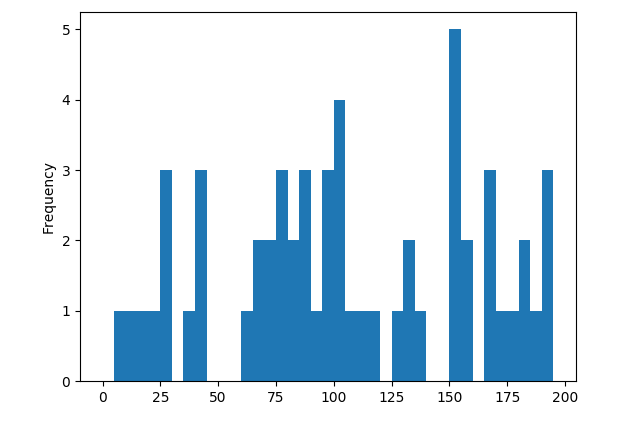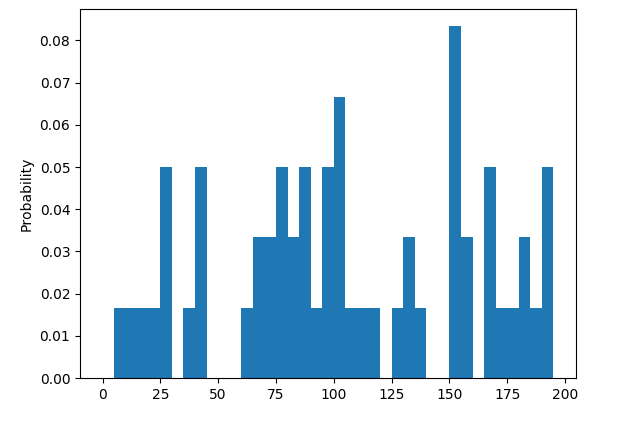第二次模拟，假设我们把车升级到当今的主流车，仪表盘的最小刻度已经到 1 了，然后时间维度上，我们细分到 0.1 分钟，那么 1 小时我们将生成 600 个数据。我们还可以进行第三次、第四次、甚至是无穷次的模拟，每次模拟的时候我们都将行驶速度的精度进一步提升、将时间间隔进一步缩小，让两者都趋近于 0，那么我们的模拟就从离散逐步趋近于连续的值了。
随机变量的概率分布由离散型的直方图变为了连续型的曲线图。通过下面的图，你可以看到这整个演变的过程。
当速度间隔和时间间隔（精度）逐步缩小的时候，直方图的分组（bin）就越小，所以你会看到 x 轴上的数据越浓密，y 轴上的数据越平滑。当间隔或精度无穷小并趋近于 0 的时候，y 轴的数据就会随着 x 轴连续变化而变化。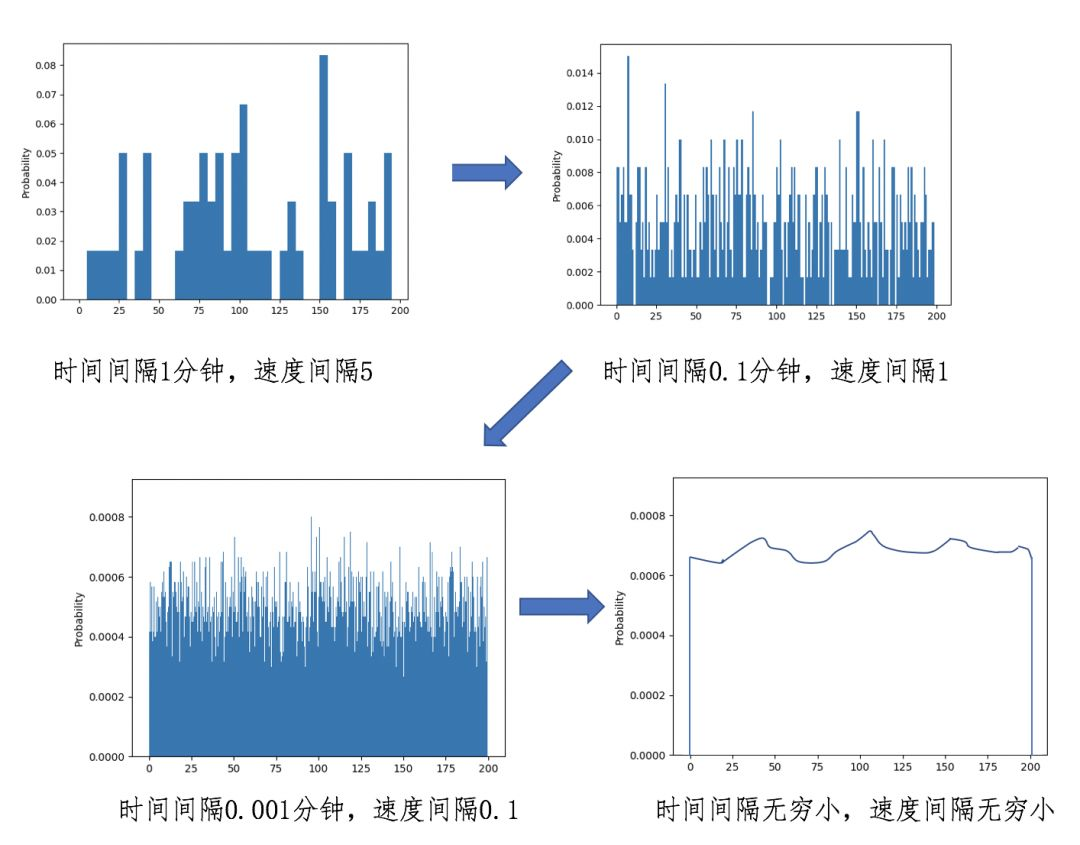不过，当时间间隔小于数秒时，我们需要考虑随机产生的数据是否具备真实性，毕竟现实中汽车的速度不可能在数秒中从 0 到 200 公里，因此临近两次的采样数据不能相差太大。
上面我通过两个模拟实验，分别展示了离散型和连续型概率的分布。其实，人们在实际运用中，已经总结出了一些概率分布，我这里挑几个最常见的给你讲解。
首先我们来看看离散分布模型。常用的离散分布有伯努利分布、分类分布、二项分布、泊松分布等等，这里我重点给你介绍两个。
第一个是伯努利分布（Bernoulli Distribution），这是单个随机变量的分布，而且这个变量的取值只有两个，0 或 1。伯努利分布通过参数λ来控制这个变量为 1 的概率，我把具体的公式列出来，并画了张图便于你理解。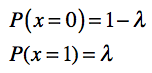或者写作：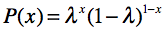其中 x 只能为 0 或 1。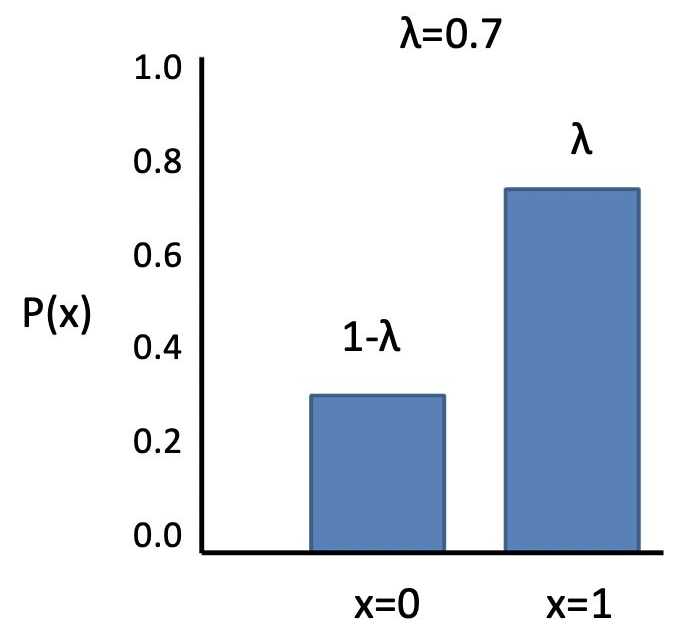从这个图也可以看出，之前抛硬币的概率分布就属于伯努利分布。
另一个是分类分布（Categorical Distribution），也叫 Multinoulli 分布。它描述了一个具有 k 个不同状态的单个随机变量。这里的 k，是有限的数值，如果 k 为 2 的时候，那么分类分布就变成了伯努利分布。我把这个分布的公式和图解都列了出来。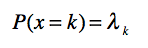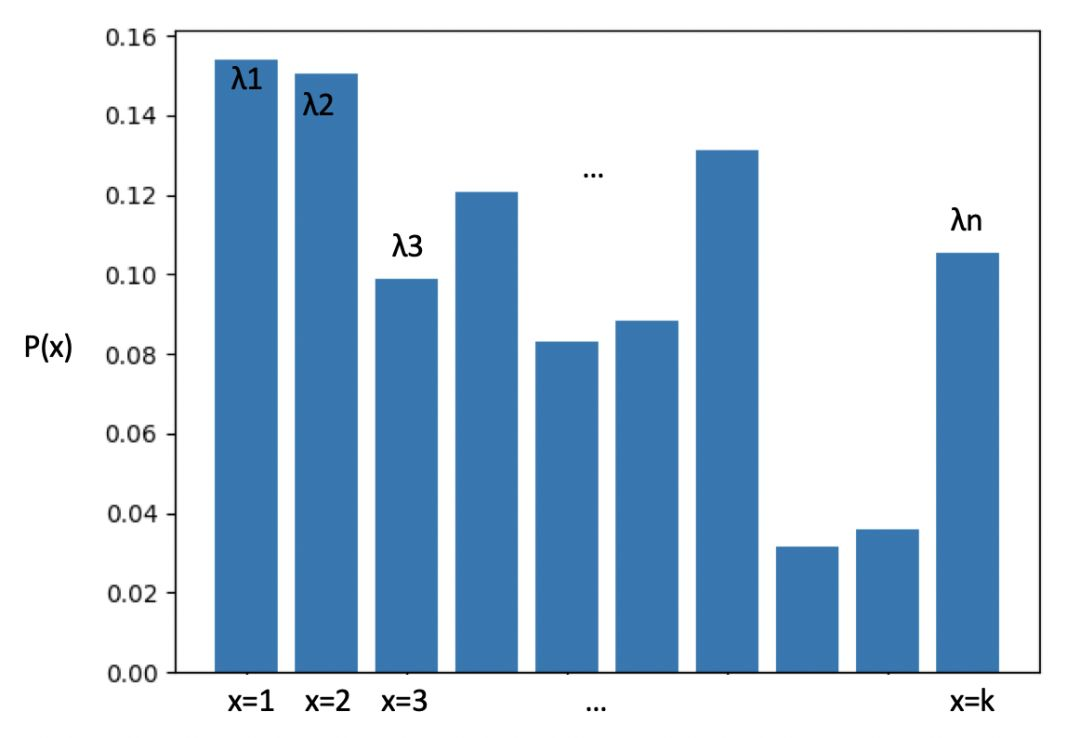离散型随机变量的状态数量是有限的，所以可以通过伯努利和分类分布来描述。可是对于连续型随机变量来说，状态是无穷多的，这时我们就需要连续分布模型。比较经典的连续分布有正态分布、均匀分布、指数分布、拉普拉斯分布等等。如果你只需要掌握一个的话，那肯定是正态分布。
这个分布可以近似表示日常生活中很多数据的分布，我们经常使用它进行机器学习的特征工程，对原始数据实施标准化，使得不同范围的数据具有可比性。所以，如果想要学习机器学习，一定要掌握正态分布。
正态分布（Normal Distribution），也叫高斯分布（Gaussian Distribution）。我把这个分布的公式列在这里：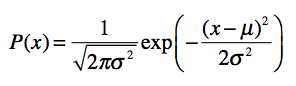在这个公式中有两个参数，μ表示均值，σ表示方差。看这个公式不太直观，我们来看一看对应的分布图。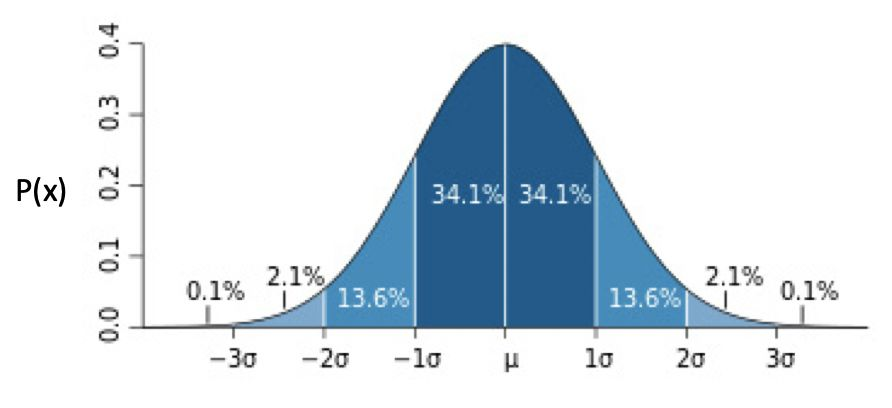从这个图可以看出，越靠近中心点μ，出现的概率越高，而随着渐渐远离μ，出现的概率先是加速下降，然后减速下降，直到趋近于 0。蓝色区域上的数字，表示了这个区域的面积，也就是数据取值在这个范围内的概率。例如，数据取值在 [-1σ, μ] 之间的概率为 34.1%。
现实中，很多数据分布都是近似于正态分布的。例如人类的身高体重。拿身高来说，大部分人都是接近平均值身高，偏离平均身高越远，相对应的人数越少。这也是为什么正态分布很常用的原因。
正态分布可以扩展到多元正态分布或多维正态分布（Multivariate Normal Distribution），不过最实用的还是一元标准正态分布，这种分布的μ为 0，σ为 1。在专栏后面的内容里，我会详细介绍如何使用这种分布，进行特征值的标准化。
期望值
理解了概率分布，你还需要了解期望值。为什么要了解期望值呢？
期望值，也叫数学期望，是每次随机结果的出现概率乘以其结果的总和。如果我们把每种结果的概率看作权重，那么期望值就是所有结果的加权平均值。它在我们的生活中十分常见，例如计算多个数值的平均值，其实就是求期望值，只不过我们假设每个数值出现的概率是相同的。
上一节，我提到如何使用概率来解决复杂度分析，通过概率的加权平均来获得平均时间复杂度，就是时间复杂度的期望值。当然，这个概念能帮助你解决的实际问题远不止这些。
在我看来，一个问题只要满足两个要素，我们就可以考虑使用期望值：
第一个要素，在这个问题中可能出现不同的情况，而且各种情况的出现满足了一定的概率分布；
第二个要素，每种情况都对应一个数值，这个数值代表了具体的应用含义。
如果你觉得这个还是过于抽象，我再回到本节的案例给你讲解。我先给你提个问题：给定了行驶速度的概率分布，如何计算汽车在 1 小时内每分钟行驶的平均速度？我们还从比较容易理解的离散型随机变量开始。
没错，这个问题的答案就是使用 1 小时所行驶的总距离除以 60 分钟。以之前的每分钟读取仪表盘、仪表盘最小刻度是 5 为例。概率分布如下图。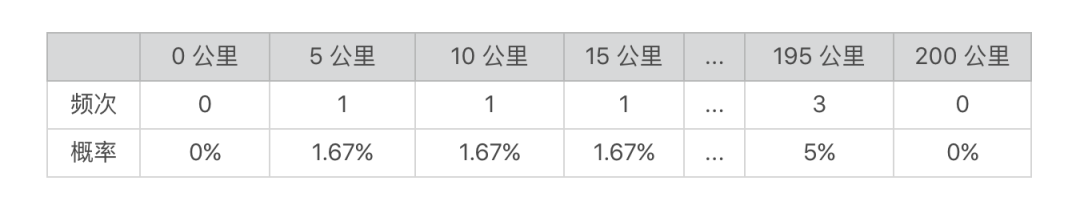1 小时行驶的总距离为每种速度乘以用该速度行驶的时间的乘积之总和。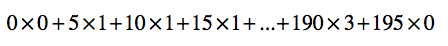1 小时内每分钟平均的行驶速度为总距离除以 60 分钟。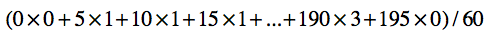将上述式子变化一下，我们可以得到：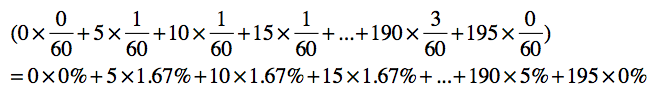你会发现，每分钟的平均值，就是每种速度的加权平均，而每种速度的权重就是其在概率分布中出现的概率。汽车可能按照不同的速度行驶，每种速度都有一个出现的概率，就是我前面提到的第一个要素。而每种速度所对应的每分钟多少公里这个数值，就是第二个要素。结合这两个要素，计算得到的平均值，也是汽车每分钟行驶速度的期望值。
那么，对于连续型的随机变量，这种期望值应该如何计算呢？我们需要使用下面的积分公式：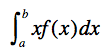总结
通过这讲的内容，你对概率的基本知识应该有所了解。本讲中，我通过抛硬币和汽车驾驶的例子，讲述了概率论中一些最基本，也是最重要的概念，包括随机现象、随机变量、概率分布和期望值。
离散型的随机变量在计算机编程中的应用更为广泛。它可以和排列组合的思想结合起来，通过不同排列或组合的数量，计算每种情况出现的概率。如果把这种概率和每种情况下的复杂度数值结合起来，就可以计算复杂度的期望值。
另外，离散型概率也可以运用在机器学习的分类算法中。例如，对于文本进行分类时，我们可以通过离散型随机变量，表示每个分类或者每个单词出现的概率。当然，仅靠今天的内容，还不足以让我们打造一个分类算法。我们还需要了解联合概率、条件概率、贝叶斯定理等重要的概念。
上一节我介绍了随机现象、随机变量以及概率分布这些比较简单的概念。学习这些概念是为了做什么呢？其实就是为了更精确地描述我们生活中的现象，用数学的视角看世界，以此解决其中的问题。
但是实际生活中的现象并非都会像“投硬币”那样简单。有很多影响因素都会影响我们去描述这些现象。比如，看似很简单的“投硬币”，我们其实只是考虑最主要的情况，粗暴地把硬币出现的情况一分为二。比如说，不同类型的硬币是否会影响正反面的概率分布呢？站立的情况如何考虑呢？再比如说，在汽车速度的例子中，经过的交通路线，不同的路线是否会影响速度的概率分布呢？
一旦影响因素变多了，我们需要考虑的问题就多了。想要解决刚才那几个问题，更精确地描述这些现象，我们就需要理解几个新的概念，联合概率、条件概率以及贝叶斯法则。从数学的角度来说，这些概念能描述现实世界中更为复杂的现象，建立更精细的数学模型。比如，我们后面要讲的朴素贝叶斯算法就是建立在联合概率、条件概率和边缘概率之上的。所以，这一节的内容也非常重要，你一定要认真学习并且掌握。
联合概率、条件概率和边缘概率
最近，我一直在操心儿子的教育问题，所以一直在研究他班级的成绩单。为了弄清我儿子在班级上的成绩排名，我向老师要了张全班成绩的分布表。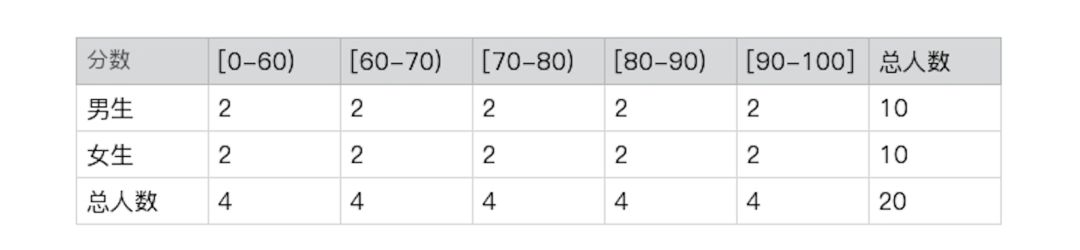这张表中有两个随机变量，一个是学生的性别，一个是分数区间。我们很容易就可以得出，这个班中男生的概率是 P(男生)=10/20=50%，90 分及以上的学生的概率是 P(90-100)=4/20=20%。那全班考了 90 分以上的男生的概率是多少呢？我们只要找到 90 分以上的男生人数，用这个人数除以全班总人数就行了，也就是 P(男生, 90-100)=2/20=10%。
你有没有发现，“90 分以上的男生”这个概率和之前单独求男生的概率或 90 分以上的概率不一样。之前只有一个决定因素，现在这个概率由性别和分数这两个随机变量同时决定。这种由多个随机变量决定的概率我们就叫联合概率，它的概率分布就是联合概率分布。随机变量 x 和 y 的联合概率使用 P(x, y) 表示。我算出了这个例子里所有的联合概率分布。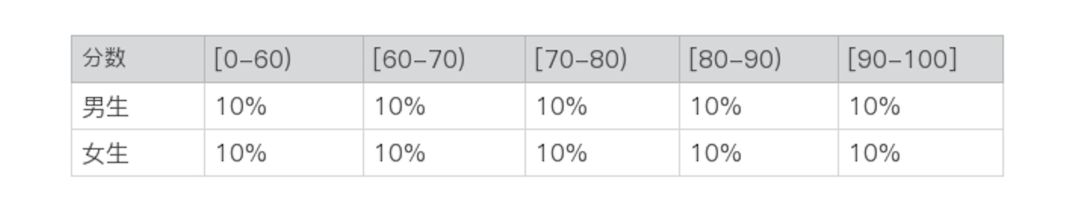这里的例子只有两个随机变量，但是我们可以很容易扩展到更多的随机变量，比如再增加一个学科的变量。那么，我们就可以观测这样的数据：“班级上女生的数学考了 90 分及以上的概率是多少？”，其中女生是关于性别变量，数学是关于学科变量，而 90 分及以上是关于分数变量。
那么联合概率和单个随机变量的概率之间有什么关联呢？对于离散型随机变量，我们可以通过通过联合概率 P(x, y) 在 y 上求和，就可以得到 P(x)。对于连续型随机变量，我们可以通过联合概率 P(x, y) 在 y 上的积分，推导出概率 P(x)。这个时候，我们称 P(x) 为边缘概率。
除了边缘概率的推导，多个变量的联合概率和单个变量的概率之间还存在一个有趣的关系。在解释这个关系之前，让我先来介绍条件概率。
条件概率也是由多个随机变量决定，但是和联合概率不同的是，它计算了给定某个（或多个）随机变量的情况下，另一个（或多个）随机变量出现的概率，其概率分布叫做条件概率分布。给定随机变量 x，随机变量 y 的条件概率使用 P(y | x) 表示。
回到成绩分布的案例。我能理解在不同的阶段，男生和女生的成绩可能无法直接相比。所以我更关心的是，自己儿子和其他男生相比是否落后了。那么我的脑子里就产生了这样一个问题：“在男生中，考 90 分及以上的概率是多少？”。
仔细看，这个问题和前面几个有所不同，我只关心男生这个群体，所以解答应该是找到考了 90 分以上的男生之人数，然后用这个人数除以男生总人数（注意，不再是全部总人数）。根据上述表格的数据来计算，P(90-100|男生)= 2/10=20%。
解释清楚了条件概率，我就可以列出概率、条件概率和联合概率之间的“三角”关系了。简单的说，联合概率是条件概率和概率的乘积，采用通用的公式来表达就是：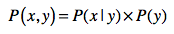同样的道理，我们也可以得到：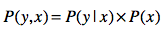我们仍然可以使用成绩的案例，来验证这个公式。为了更清晰地表述这个问题，我们使用如下的符号：
|男, 90-100|表示考了 90 分以上的男生人数；
|男|表示男生人数；
|全班|表示全班人数。
男生中考了 90 分及以上的概率为 P(90-100 | 男生) = |男生, 90-100| / |男生|，全班中男生的概率为 P(男) = |男生| / |全班|。如果我们将 p(90-100 | 男生) 乘以 P(男) 会得到什么结果呢？
(|男, 90-100| / |男生|) * (|男生| / |全班|) = |男, 90-100| / |全班|
咦，这不就是全班中男生考了 90 分及以上的联合概率吗？其实，概率、条件概率和联合概率之间的这种“三角”关系，也是著名的贝叶斯定理的核心，下面我来详细解释什么是贝叶斯定理，以及它可以运用在什么场景之中。
贝叶斯定理
我们假设有这样一个场景，我想知道男生考了 90～100 分的概率有多少，来评估一下儿子在男生中算什么水平。可是老师出于隐私保护，并没有把全班数据的分布告诉我，她说道“我可以告诉你全班考 90～100 分的概率，以及 90～100 分中男生的概率，但是不能告诉你其他信息了”。这个时候，贝叶斯定理就可以帮上忙啦。
刚刚我们提到：所以就有：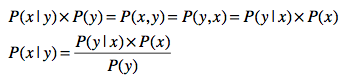这就是非常经典的贝叶斯法则。为什么说经典呢？是因为它有很多应用的场景，比如朴素贝叶斯，你可以多多熟悉一下这个公式。在这个公式中，还包含了先验概率（Prior Probability）、似然函数（Likelihood）、边缘概率（Marginal Probability）和后验概率（Posterior Probability）的概念。
在这里面，我们把 P(x) 称为先验概率。之所以称为“先验”，是因为它是从数据资料统计得到的，不需要经过贝叶斯定理的推算。
P(y | x) 是给定 x 之后 y 出现的条件概率。在统计学中，我们也把 P(y | x) 写作似然函数 L(x | y)。在数学里，似然函数和概率是有区别的。概率是指已经知道模型的参数来预测结果，而似然函数是根据观测到的结果数据，来预估模型的参数。不过，当 y 值给定的时候，两者在数值上是相等的，在应用中我们可以不用细究。
另外，我们没有必要事先知道 P(y)。P(y) 可以通过联合概率 P(x, y) 计算边缘概率得来，而联合概率 P(x, y) 可以由 P(y|x) * P(x) 推出。针对离散型和连续型的边缘概率推导分别如下：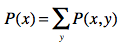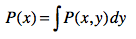而 P(x|y) 是根据贝叶斯定理，通过先验概率 P(x)、似然函数 P(y | x) 和边缘概率 P(y) 推算而来，因此我们把它称作后验概率。
回到刚刚的案例，我可以通过这样的式子来计算男生考 90-100 分的概率：
P(90-100 | 男生) = (P(男生 | 90-100) * P(90 -100)) / P(男生)
我只需要数数看，班上男生有多少、总人数多少，就能算出 P(男生)，或者也可以使用 P(男生 | 90-100) 和 P(90-100) 算出边缘概率 P(男生)。在加上之前，老师告诉我的 P(男生 | 90-100) 和 P(90 -100)，就能推算出 P(90-100 | 男生) 了。这个例子就是通过先验概率，推导出后验概率，这就是贝叶斯定理神奇的地方，也是它最主要的应用场景。
随机变量之间的独立性
说到多个随机变量的联合和条件概率，你可能会产生一个问题：这些随机变量是否会相互影响呢？比如，性别和分数之间有怎样的关系？性别是否会影响分数的概率分布？在之前的成绩分布表中，我们可以得到：
p(90-100 | 男生) = 20%
p(90-100 | 女生) = 20%
p(90-100) = 20%
所以，p(90-100 | 男生) = p(90-100 | 女生) = p(90-100)，也就是全班中考 90 分及以上的概率、男生中考 90 分及以上的概率、以及女生中考 90 分及以上的概率，这三者都是一样。以此类推到其他的分数区间，同样如此，那么，从这个数据上得出的结论是性别对考分的区间没有影响。反之，我们也可以看到 p(男生 | 90-100) = p(男生 | 80-90) = p(男生 | 70-80) = … = p(男生) = 50%，也就是说考分区间对性别没有影响。这种情况下我们就说性别和分数这两个随机变量是相互独立的。
相互独立会产生一些有趣的现象，刚刚我们提到：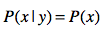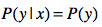另外，将 p(x | y) = p(x) 带入贝叶斯公式，就可以得出：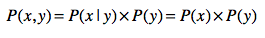变量之间的独立性，可以帮我们简化计算。举个例子，假设有 6 个随机变量，而每个变量有 10 种可能的取值，那么计算它们的联合概率 p(x1, x2, x3, x4, x5, x6)，在实际中是非常困难的一件事情。
根据排列，可能的联合取值，会达到 10 的 6 次方，也就是 100 万这么多。那么使用实际的数据进行统计时，我们也至少需要这个数量级的样本，否则的话很多联合概率分布的值就是 0，产生了数据稀疏的问题。但是，如果假设这些随机变量都是相互独立的，那么我们就可以将联合概率 p(x1, x2, x3, x4, x5, x6) 转换为 p(x1) * p(x2) * p(x3) * p(x4) * p(x5) * p(x6)。如此一来，我们只需要计算 p(x1) 到 p(x6 ) 就行了。
不过，班主任刚刚打电话给我，说之前的数据表格有点笔误，实际的分布应该是这样的：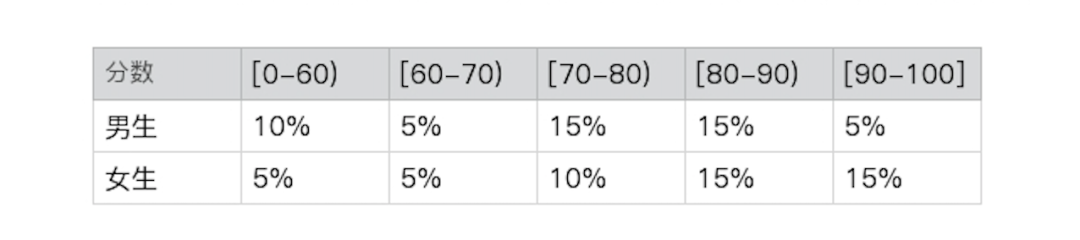你再推算一下，就会发现 p(分数 | 性别) = p(分数) 和 p(性别 | 分数) = p(性别) 并不成立，所以两者不再是相互独立的。所以下面这个式子就不再成立了，这点你在计算的时候需要注意。在实际项目中，我们会假设多个随机变量是相互独立的，并基于这个假设大幅简化计算，降低对数据统计量的要求。虽然这个假设通常是不成立的，但是仍然可以帮助我们得到近似的解。相比较实现的可行性和求解的精确度，可行性更为重要。在讲解朴素贝叶斯方法中，我会充分利用这一点，从有限的训练样本中构建分类器。
总结
这一讲我继续讨论了概率分布相关的内容，不过这次的重点是多个随机变量相关的联合概率、条件概率、边缘概率。这里概念有点多，但是都很重要，因为这三者之间的推算关系，直接构成了贝叶斯定理的核心，所以你要花点时间理解并记住它们。
而贝叶斯定理定义了先验概率、后验概率和似然函数，后验概率和似然函数及先验概率的乘积成正比关系。此外，通过多个变量之间的独立性，我们可以简化联合概率的计算问题。贝叶斯定理和变量之间独立性的假设，对后面理解朴素贝叶斯算法很有帮助。
如果有一定数量的标注数据，那么通过统计的方法，我们可以很方便地得到先验概率和似然函数，然后推算出后验概率，最后依据后验概率来做预测。这整个过程符合监督式机器学习的模型训练和新数据预测这两个阶段，因此朴素贝叶斯算法被广泛运用在机器学习的分类问题中。下一节中，我们详细讨论这个算法。
概率基础：节选自极客时间专栏（程序员的数学基础），作者黄申。备注：公众号菜单包含了整理了一本AI小抄，非常适合在通勤路上用学习。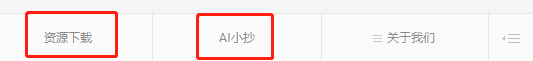往期精彩回顾

2019年公众号文章精选适合初学者入门人工智能的路线及资料下载机器学习在线手册深度学习在线手册AI基础下载（第一部分）备注：加入本站微信群或者qq群，请回复“加群”加入知识星球（4600+用户，ID：92416895），请回复“知识星球”

喜欢文章，点个在看展开全文• ## 多元正态分布的条件概率分布(一)

万次阅读 多人点赞 2014-06-04 12:25:20
多元正态分布的条件概率分布 假设分别有两个多维向量和 其中 那么的协方差矩阵为： 那么的协方差矩阵为： 那么的协方差矩阵为： 那么的协方差矩阵为...
多元正态分布的条件概率分布

假设分别有两个多维向量和

其中

那么的协方差矩阵为：

那么的协方差矩阵为：

那么的协方差矩阵为：

那么的协方差矩阵为：

那么向量的协方差矩阵为

其中

其中

那么条件分布为

其中

现在来看一个二维正态分布的例子
均值为
协方差矩阵为

令他们都只包含一个元素

那么条件分布

我们来看条件均值

这里
将其代入条件均值表达式得到

同样得到，条件协方差矩阵

我们可以得到条件分布为

同样可以得到

如果代入具体的数值，我们可以得到

那么给定标准正态分布

证明：

设F(y)为f(x)的累积分布,那么   令  那么

那么
如果从标准正态分布采样任意均值方差的公式就可以得到了

证明过程如下

令     F(y)是f(y)的累积分布，那么            那么

对二元正态分布的条件分布进行采样就可以转变成对一维正态分布的采样

同理可以得到X1条件下的采样方法。

对于Gibbs采样的情况，我们需要考虑k维正态分布的条件概率
我们假定X1为一维变量，X2为k-1维的向量

可以看出，在K维条件下，还是需要求出k-1维的逆矩阵，这个计算量同样不小，这方面的问题留在后面来解决

展开全文• 《统计学习方法》中关于多元逻辑回归公式推导，写的比较简单，正好前几天有同事对此比较疑惑，因此，在此进行详细推导，有助于大家共同学习。 首先，关于逻辑回归中二分类问题: P(Y=1∣x)=exp(ω⋅x+b)1+exp(ω⋅x+b...
• 条件概率条件概率表示在条件Y=b成立的情况下，X=a的概率，记作P(X=a|Y=b)或P(a|b)，它具有如下性质：“在条件Y=b下X的条件分布”也是一种“X的概率分布”，因此穷举X的可取值之后，所有这些值对应的概率之和为1即...高斯分布
• 概率的乘法公式： 设A，B为随机试验E上两个事件，且P(A)，则有 。 0x2：事件的相互独立性 在一个随机试验中，A，B是两个事件。如果它们之间是否发生是相互不影响的，这表现为 P(B | A) = P (B) 或 P(A |...
• 即，如何证明多元高斯的条件分布和边际分布也是高斯，并给出其形式。 首先，我们知道，一个均值为μ\muμ、协方差矩阵为Σ\SigmaΣ的多元正态分布的概率密度函数可以表示为 1(2π)k/2∣Σ∣1/2exp(−12(x−μ)TΣ−...机器学习 矩阵
• 服从正态分布且样本之间独立同分布，由以上的推导可以得到类条件概率密度 p ( x | y ) p ( x | y ) p(x \vert y) 的MLE： μ ^ y = 1 n y ∑ i : y i = y x i (1) (1) μ ^ y = 1 n y ∑ i : y i = y x i \hat ...
• 一、推导过程: 二、结果： 边缘分布 x1,x2 各自依然服从 μi，写反差矩阵 Σii 的多元高斯分布；...条件概率分布 给定 xj 求 xi 的分布： μi|j=μi+ΣijΣ−1jj(xj−μj) Σi|j=Σjj−ΣTijΣ−1iiΣij ...机器学习
• 在看论文的时候看到这样一个公式： 注意这个式子里面的位置。 我的第一反应是，这不对吧？思前想后，我决定推一推试试看。...考虑一种简单的情况，如果事件与事件和事件是独立的...等式左边直接用条件概率公式展开：贝叶斯
• 这些随机变量的取值是由试验结果确定的，因此它们的取值会存在相互关联，这里我们先以离散型随机变量为例，将离散型随机变量的分布列和期望推广到多个随机变量的情况，并且进一步在此基础上讨论多元随机变量条件和...机器学习 大数据 人工智能 python
• 各类别总体的概率分布是已知的 要决策分类的类别数是一定的 简单说，就是我们知道有多少个类别，以及每一个类别的概率值 d维特征向量 在连续情况下，假设要识别的对象有d种特征量x1，x2，…，xd，这些特征的...
• 高斯那些公式 已知 D 维向量 x，其高斯概率分布为： N(x|μ,Σ)==1(2π)D/21|Σ|1/2exp(−12(x−μ)TΣ−1(x−μ))1|Σ|(2π)D−−−−−−−√exp(−12(x−μ)TΣ−1(x−μ)) 显然默认 x 是一个列向量 还需...
• 在前面两节中，我们介绍了多元随机变量的有关概念，重点围绕着多元随机变量的联合概率条件与边缘概率分布以及独立性和相关性，阐述了多元随机变量之间的关系，这些都是多元随机变量重点需要关注和研究的问题。...可视化 python 机器学习 人工智能 数据分析
• p(a|b)：等同于P(X=a|Y=b)，在条件 Y=b 成立的情况下，X=a 的概率 p(a,b)：等同于P(X=a,Y=b)，X=a，Y=b 状态下的概率——联合概率 p(ab)：等同于 p(a,b) 注意点： 区分几个概念：”随机变量”A和“随机变量的...
• 推论为本人学完第九周后自己推导得出，推论公式在文中下半部分。本来在word上编辑好了公式复制到博客上乱码了，所以有些公式是粘贴的图片，不影响观看。欢迎大家指正，交流。 在一般的高斯分布模型中，我们计算高斯...吴恩达
• 概率,期望，方差 　只有一个变量时 　F(x<=a) =∫-∞af(x)dx 　当区间取负无穷到正无穷时积分为1 　推广到多元之后: 　 　同理，当区间取满整个空间时，积分为1 　f被称为概率密度函数 　边缘分布函数...
• 多元高斯分布中有一条重要的性质，如果两个变量集的联合是高斯分布，那么其中一个变量集在以另一个变量集为条件下的分布依然是高斯分布，并且可以通过公式推导求出该条件的期望和方差。下面给出具体分析。 假设D维...高斯分布
• clear all; close all; clc; %randn('seed',0); %%一维高斯函数 mu=0; sigma=1; x=-6:0.1:6; y=normpdf(x,mu,sigma); plot(x,y); figure; %%二维或多维高斯函数 ...[x y]=meshgrid(linspace(-8,8,80
• 一元线性回归 回归分析只涉及到两个变量的，称一元回归分析。...多元线性回归 注：为使似然函数越大，则需要最小二乘法函数越小越好 线性回归中为什么选用平方和作为误差函数？假设模型结果与测...
• 这一小节，我们开始讨论多元随机变量之间的关系，重点围绕独立性和相关性的概念展开。 1.关于独立性的讨论 1.1.随机变量与事件的独立性 ...如果用条件概率的式子来表示的话，就有： 如果随机变量XXX独立于事件协方差 机器学习 人工智能 大数据 数据分析
• 原链接 ...阅读目录(Content) 从贝叶斯方法（思想）说起...0x1：条件概率公式 0x2：全概率公式和贝叶斯概率公式 0x3：随机变量的链式法则（chain rule） 0x4：随机变量的独立性 0x5：随机变量条件独立性 0x6：查询一个...
• 一维正态分布推广到多维正态分布推导过程中会加入推导所必需的理论从一维标准正态分布说起， ，其概率密度函数为 二维标准正态分布的概率密度函数为： 维标准正态分布的概率密度函数为：（其中 ） 接下来我们需要将......